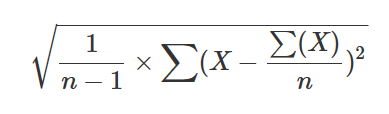# stdev() (aggregation function)

Calculates the standard deviation of Expr across the group, using Bessel's correction for a small data set that is considered a sample.

For a large data set that is representative of the population, use stdevp() (aggregation function).

• Used formula:• Can be used only in context of aggregation inside summarize

## Syntax

`stdev` `(`Expr`)`

## Arguments

• Expr: Expression that will be used for aggregation calculation.

## Returns

The standard deviation value of Expr across the group.

## Examples

``````range x from 1 to 5 step 1
| summarize make_list(x), stdev(x)

``````
list_x stdev_x
[ 1, 2, 3, 4, 5] 1.58113883008419